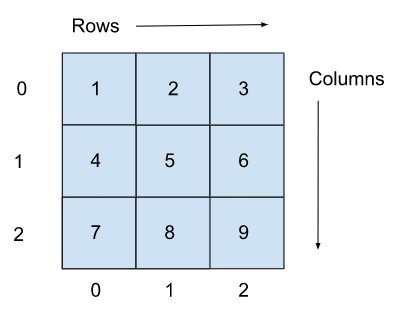# Golang program to print a 2D array?

Two−dimensional arrays are commonly used in Go. For example, storing data in a table requires a 2D array. In this tutorial, we will be discussing the approach to printing a 2D array in Golang programming.

But before writing this code, let’s briefly discuss the two−dimensional array.

## What is a 2D Array?

A 2D array is comprised of rows and columns. Its nature is similar to a normal array with a fixed length. It is homogeneous in nature, i.e., all the elements stored in a 2D array should be of the same data type.

If a 2D array has ‘r’ rows and ‘c’ columns, then the first element in an array is stored at the (0,0) index and the last element is located at the (r−1,c−1) index.

### Example

• rows = 3, columns = 3

This means the array has nine elements then−

• First value of the array will be at the (0,0) index, and

• Last value will be at the (row−1,column−1) index, i.e. (2,2) index.## Example 1: Printing the 2D array with numbers from 1 to 9.

### Algorithm

• Step 1 − Declare a multi-dimension array ‘arr’ with few elements stored in it.

• Step 2 − Create a for loop starting from 0 (as array indexing starts from 0) and run it until it is less than the number of rows.

• Step 3 − Create a nested for loop starting from 0 and run it until it is less than the number of columns.

• Step 4 − Print the array elements.

### Example

This program will print the 2D array with 3 rows and 3 columns. This 2D array stores numbers from 1 to 9.

// Go program to print a multi-dimension array
package main

// fmt package allows us to print formatted strings
import "fmt"

func main() {
// an array with 3 rows and 3 columns
arr := int{
{1, 2, 3},
{4, 5, 6},
{7, 8, 9}}

// Accessing the values of the array using nested for loop
fmt.Println("Elements of Array:")
for i := 0; i < 3; i++ {
for j := 0; j < 3; j++ {
// printing the array elements
fmt.Printf("arr[%d][%d] = %d\n", i, j, arr[i][j])
}
}
}


### Output

Elements of Array:
arr = 1
arr = 2
arr = 3
arr = 4
arr = 5
arr = 6
arr = 7
arr = 8
arr = 9


## Example 2: Printing the 2D array which stores Book name and its Author

### Algorithm

• Step 1 − Declare a multi−dimension array ‘arr’ with few elements stored in it.

• Step 2 − Create a for loop starting from 0 (as array indexing starts from 0) and run it until it is less than the number of rows.

• Step 3 − Create a nested for loop starting from 0 and run it until it is less than the number of columns.

• Step 4 − Print the array elements.

### Example

This program will print the 2D array with 5 rows and 2 columns. This 2D array stores the book name and its respective author and prints this information.

// Go program to print a two-dimensional array
package main

// fmt package allows us to print formatted strings
import "fmt"

func main() {
// a 2D array with 5 rows and 2 columns
arr := string{
{"Harry Potter", "J.K. Rowling"},
{"Goosebumps", "R. L. Stine"},
{"Last Heroes", "P. Sainath"},
{"The Crossing", "Jason Mott"},
{"Rain in the Mountains", "Ruskin Bond"}}

// Accessing the values of the array using for loop
fmt.Println("Elements of Array:")
for i := 0; i < len(arr); i++ {
// printing the array elements
fmt.Printf("%s was written by %s.\n", arr[i], arr[i])
}
}


### Output

Elements of Array:
Harry Potter was written by J.K. Rowling.
Goosebumps was written by R. L. Stine.
Last Heroes was written by P. Sainath.
The Crossing was written by Jason Mott.
Rain in the Mountains was written by Ruskin Bond.


### Description of code

• for i := 0; i < len(arr); i++ − Here, we are using the for loop for traversing the rows of the two−dimensional array. The loop is starting from 0 and will run until the value of i < len(arr). The length of the array is getting fetched using the ‘len’ function

• fmt.Printf("%s was written by %s.\n", arr[i], arr[i]) − We are printing arr[i] as in the first column the book name is present and then we are printing arr[i] as the author name is present in the second column of the array.

## Conclusion

This is all about printing the elements of a two−dimensional array using two examples. Two−dimensional arrays play a great role in many real−world scenarios. You can explore more about Golang programming using thesetutorials.

Updated on: 28-Dec-2022

677 Views